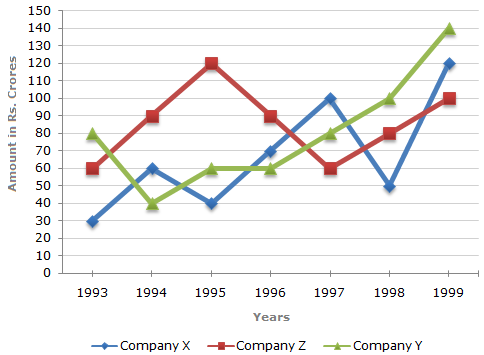# Data Interpretation - Line Charts - Discussion

Discussion Forum : Line Charts - Line Chart 1 (Q.No. 1)
Directions to Solve

Study the following line graph and answer the questions.

Exports from Three Companies Over the Years (in Rs. crore)1.
For which of the following pairs of years the total exports from the three Companies together are equal?
1995 and 1998
1996 and 1998
1997 and 1998
1995 and 1996
Explanation:

Total exports of the three Companies X, Y and Z together, during various years are:

In 1993 = Rs. (30 + 80 + 60) crores = Rs. 170 crores.

In 1994 = Rs. (60 + 40 + 90) crores = Rs. 190 crores.

In 1995 = Rs. (40 + 60 + 120) crores = Rs. 220 crores.

In 1996 = Rs. (70 + 60 + 90) crores = Rs. 220 crores.

In 1997 = Rs. (100 + 80 + 60) crores = Rs. 240 crores.

In 1998 = Rs. (50 + 100 + 80) crores = Rs. 230 crores.

In 1999 = Rs. (120 + 140 + 100) crores = Rs. 360 crores.

Clearly, the total exports of the three Companies X, Y and Z together are same during the years 1995 and 1996.

Discussion:
9 comments Page 1 of 1.

Peter said:   2 years ago
1993 = Rs. (30 + 80 + 60) cr = Rs. 170 cr
1994 = Rs. (60 + 40 + 90) cr = Rs. 190 cr.
1995 = Rs. (40 + 60 + 120) cr= Rs. 220 cr.
1996 = Rs. (70 + 60 + 90) cr= Rs. 220 cr
1997 = Rs. (100 + 80 + 60) cr = Rs. 240 cr
1998 = Rs. (50 + 100 + 80) cr= Rs. 230 cr
1999 = Rs. (120 + 140 + 100) cr= Rs. 360 cr
Since in 1995 and 1996 export = 220 cr.

Jaaneman said:   6 years ago
First visualize the question.

Given explanation is better to solve the problem. Once try it.

Yenkaayamma said:   7 years ago
Is there any easy way to solve?

Subbalakshmi subbi said:   7 years ago
Hai guys how do you do?

Itaf Hussain said:   9 years ago
Here is the way for solving this problem in less time.

Just look at the four options available you will notice there are only four years i.e. 1995, 1996, 1997, 1998.

So Just calculate the total exports for the following four years 1995, 1996, 1997, 1998.

Humera said:   9 years ago
Can't it able to calculate by an easy method to manage our time.

Raviraj said:   9 years ago
Is there any easy way to calculate within a few seconds?